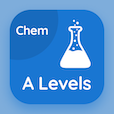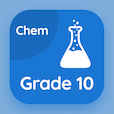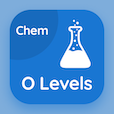Online A Level Courses

A Level Chemistry Practice Tests

A Level Chemistry Online Tests

The Book Amount of Substance Multiple Choice Questions (MCQ Quiz) with answers, Amount of Substance MCQ Quiz PDF download to study online a level chemistry courses. Practice Moles and Equations Multiple Choice Questions and Answers (MCQs), Amount of Substance quiz answers PDF for best online colleges for teaching degree. The eBook Amount of Substance MCQ App Download: solutions and concentrations, gas volumes, mole calculations, chemical formula and equations test prep for GRE subject tests.

The MCQ: Number of moles of substance can be determined by PDF, "Amount of Substance" App Download (Free) with mass of substance/molar mass, mass of substance/molecular mass, atomic weight of substance/avogadro constant, and volume of substance choices for best online colleges for teaching degree. Study amount of substance quiz questions, download Google eBook (Free Sample) for GRE subject test tutoring.

## Chemistry MCQs: Amount of Substance Quiz Questions

MCQ: Number of moles of substance can be determined by

A) mass of substance/molar mass
B) mass of substance/molecular mass
C) atomic weight of substance/Avogadro constant
D) volume of substance

MCQ: Avogadro's constant is the number

A) 6.02 x 1023
B) 6.03 x 1032
C) 1.2 x 106
D) 1.23 x 10 62

MCQ: Avogadro's constant is the number which was first calculated by

A) Joseph Loschmidt
C) Galileo
D) Charles

MCQ: The symbol used to denote Avogadro's constant is

A) A
B) L
C) V
D) G

MCQ: gmol-1 is the standard unit for

A) molecular mass
B) molar mass
D) atomic mass

### Practice Tests: A Level Chemistry Exam Prep

Download A level Chemistry Quiz App, 10th Grade Chemistry MCQ App, and O Level Chemistry MCQs App to install for Android & iOS devices. These Apps include complete analytics of real time attempts with interactive assessments. Download Play Store & App Store Apps & Enjoy 100% functionality with subscriptions!